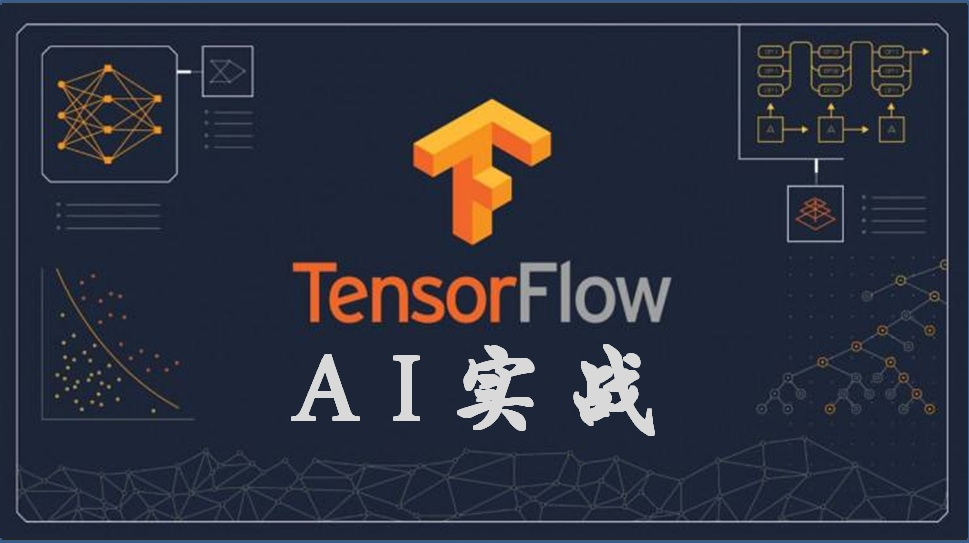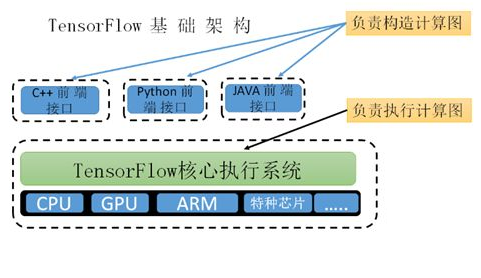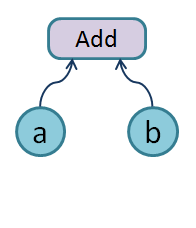# 【AI实战】快速掌握TensorFlow（二）：计算图、会话

2018/08/20 00:171、图（Graph）
TensorFlow Python库已经有一个默认图 (default graph)，如果没有创建新的计算图，则默认情况下是在这个default graph里面创建节点和边。import tensorflow as tf
a=tf.constant([1.0,2.0], name='a')
b=tf.constant([3.0,4.0], name='b')
result = tf.add(a,b)

2、会话（Session）

（1）创建一个会话

#启动默认图
sess=tf.Session()
result_value = sess.run(result)
print(result_value)
# ==> [4.0 6.0]

# 任务完成, 关闭会话.
sess.close()

（2） 创建一个会话
Session在使用完后需要关闭以释放资源，除了显式调用close外，也可以使用“with”代码块 来自动完成关闭动作。代码如下：

with tf.Session() as sess:
result_value = sess.run(result)
print(result_value)
# ==> [4.0 6.0]

（3）创建一个默认的会话

sess=tf.Session()
with sess.as_default():
result_value = result.eval()
print(result_value)

（4）创建一个交互式会话

sess= tf.InteractiveSession()
result_value = result.eval()
print(result_value)
sess.close()

3、构建多个计算图

# 构建计算图g1
g1=tf.Graph()
with g1.as_default():
# 在计算图g1中定义变量'v',并设置初始值为0。
v=tf.get_variable('v',initializer=tf.zeros_initializer()(shape = ))

# 构建计算图g2
g2=tf.Graph()
with g2.as_default():
# 在计算图g2中定义变量'v',并设置初始值微1。
v=tf.get_variable('v',initializer=tf.ones_initializer()(shape = ))

# 在计算图g1中读取变量'v'的取值
with tf.Session(graph=g1) as sess:
tf.global_variables_initializer().run()
with tf.variable_scope('',reuse=True):
print(sess.run(tf.get_variable('v')))
# 输出结果[0.]

# 在计算图g2中读取变量'v'的取值
with tf.Session(graph=g2) as sess:
tf.global_variables_initializer().run()
with tf.variable_scope('',reuse=True):
print(sess.run(tf.get_variable('v')))
# 输出结果[1.]。

4、指定运行设备

（1）在图中指定运行设备

g=tf.Graph()
# 指定计算运行的设备。
with g.device('/gpu:0'):
result=tf.add(a,b)

（2）在会话中指定运行设备

with tf.Session() as sess:
with tf.device("/gpu:0"):
result=tf.add(a,b)

• "/cpu:0": 机器的 CPU
• "/gpu:0": 机器的第一个 GPU，如果有的话
• "/gpu:1": 机器的第二个 GPU，以此类推### 作者的其它热门文章

0
7 收藏

1 评论
7 收藏
0